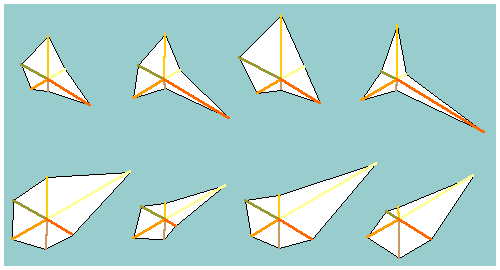less detail

#### 4.4 Exploratory Data Analysis

Visualizing numerical information is an alternative archaeological research method. It is the essence of Exploratory Data Analysis (EDA) (Tukey 1977). Instead of reducing all variations in the distribution of, for instance, the length of flint axes to two mathematical parameters (average and standard deviation), or capturing the irregularity of a site distribution pattern in a single mathematical trend surface, here the actual distribution is judged visually. The distribution of the length of axes can be simply displayed in a well-chosen histogram or a box-and-whiskers plot. Peaks or a skewed distribution will then not disappear in the 'statistical uniformity' of the normal distribution underlying the average and standard deviation. Remarkably, the exceptional observations which are often lost in the average quite set the scene, archaeologically.

The increase in computing power and growing potential of graphic software, among them Geographical Information Systems, allow a rapid and dynamic visual inspection and description of data. By continuously displaying the data in numerous diagrams and distribution maps in slightly different ways we may be able to discern structure. This is also true for multivariate data, for which the well-known Tukey stars are available, for instance. The star-shaped diagrams provide a means of visualizing the extensive numerical information. No mathematical order is calculated nor is a statistical reduction of the number of variables aimed at, but intuitively there grows an insight into the meaning of the results obtained. An insight that is also much easier to convey to students or colleagues than the 'complex' results of multivariate statistics.Fig. 38 Examples of Tukey stars